### Home > CALC > Chapter 8 > Lesson 8.3.2 > Problem8-118

8-118.
1. Determine the following limits. Homework Help ✎

1.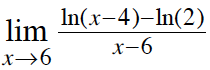2.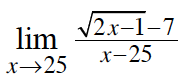3.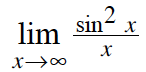4.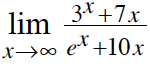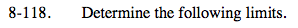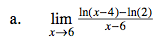This is Ana's Definition of the Derivative.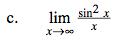For limits to infinity, we can compare the numerator with the denominator. As the numerator approaches infinity, y = sin²x oscillates between −1 and +1... not very big. As the denominator approaches infinity, y = x also approaches infinity. So the denominator is MUCH larger than the numerator.

$=\lim_{x\rightarrow \infty }\frac{\text{small}}{\text{big}}=0$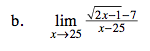See the hint in part (a).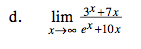Recall that the number e is about 2.718. It's larger than 2 and smaller than 3. Use that to compare the power of the numerator and denominator.